Next: Worked example 2.1: Velocity-time Up: Motion in 1 dimension Previous: Motion with constant acceleration

## Free-fall under gravity

Galileo Galilei was the first scientist to appreciate that, neglecting the effect of air resistance, all bodies in free-fall close to the Earth's surface accelerate vertically downwards with the same acceleration: namely,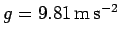.1The neglect of air resistance is a fairly good approximation for large objects which travel relatively slowly (e.g., a shot-putt, or a basketball), but becomes a poor approximation for small objects which travel relatively rapidly (e.g., a golf-ball, or a bullet fired from a pistol).

Equations (21)-(23) can easily be modified to deal with the special case of an object free-falling under gravity: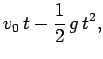(24)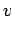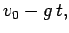(25)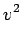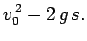(26)

Here,is the downward acceleration due to gravity,is the distance the object has moved vertically between timesand(if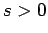then the object has risenmeters, else if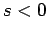then the object has fallen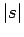meters), and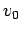is the object's instantaneous velocity at. Finally,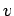is the object's instantaneous velocity at time.

Let us illustrate the use of Eqs. (24)-(26). Suppose that a ball is released from rest and allowed to fall under the influence of gravity. How long does it take the ball to fall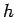meters? Well, according to Eq. (24) [with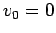(since the ball is released from rest), and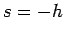(since we wish the ball to fallmeters)],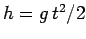, so the time of fall is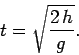(27)

Suppose that a ball is thrown vertically upwards from ground level with velocity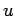. To what height does the ball rise, how long does it remain in the air, and with what velocity does it strike the ground? The ball attains its maximum height when it is momentarily at rest (i.e., when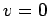). According to Eq. (25) (with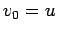), this occurs at time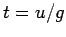. It follows from Eq. (24) (with, and) that the maximum height of the ball is given by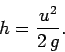(28)

When the ball strikes the ground it has traveled zero net meters vertically, so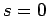. It follows from Eqs. (25) and (26) (withand) that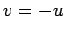. In other words, the ball hits the ground with an equal and opposite velocity to that with which it was thrown into the air. Since the ascent and decent phases of the ball's trajectory are clearly symmetric, the ball's time of flight is simply twice the time required for the ball to attain its maximum height: i.e.,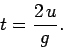(29)Next: Worked example 2.1: Velocity-time Up: Motion in 1 dimension Previous: Motion with constant acceleration
Richard Fitzpatrick 2006-02-02